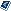MapTools.org# [maplab-users] class and style changes in Mapscript 37

Lochner,Chris [NCR] Chris.Lochner@ec.gc.ca
Mon, 14 Apr 2003 11:07:19 -0400

```This message is in MIME format. Since your mail reader does not understand
this format, some or all of this message may not be legible.

------_=_NextPart_001_01C30297.8B56BAA0
Content-Type: text/plain;
charset="iso-8859-1"

Hi Folks,

I've been having great difficulty using the new style functions in Mapscript
37.

I'm trying to extract a class object from the mapfile and change the symbol
size and color in php.

I have tried various adaptations of the mapscript 36 method but they are
quite dissimilar due to the new "style-type" instructions that 37 uses. I've
been working off the manual that Danielle (Morrisette) wrote for 37.

Here is part of the code, there are lots of different ideas in here from
other versions of mapscript but none of them seem to work,

\$class = ms_newClassObj(\$layer);
//\$class = \$layer->getClass(0);
//printf("classname = %s",\$class->name);
//printf("symbol under class =
%s",\$class->get("symbol"));
//\$class->set("symbol", "square");
\$style = ms_newStyleObj(\$class);
\$style->color->setRGB(\$rgb[r], \$rgb[g], \$rgb[b]);
//\$class->set("color", \$colorId);
\$style->size = 1;
\$style->symbol = 6;
//\$class->set("size", 4);
if (\$HTTP_FORM_VARS["CURRENT_PROG_ID"] == \$ProgramIds[\$iRow]||
(\$HTTP_FORM_VARS["CURRENT_PROG_ID"] <= 0 &&
\$HTTP_FORM_VARS["CURRENT_PROG_ID2"] == \$ProgramIds[\$iRow]))
{
\$style->symbol = 2;
\$style->size = 1;
//\$class->set("size", 8);
}
else if (\$ProgramCategory[\$iRow] == 2) {
\$style->symbol = 4;
//\$class->set("symbol", 4);
}
else {
\$style->symbolname = "star";
//\$class->set("symbol", 3);
}
\$style->outlinecolor->setRGB(0, 0, 0);

Am I on the right track with any of these? Any tips would be appreciated.

Cheers,
Chris Lochner
Lochner inc,
(Environment Canada - Water Quality Branch)

------_=_NextPart_001_01C30297.8B56BAA0
Content-Type: text/html;
charset="iso-8859-1"
Content-Transfer-Encoding: quoted-printable

<!DOCTYPE HTML PUBLIC "-//W3C//DTD HTML 3.2//EN">
<HTML>
<META HTTP-EQUIV=3D"Content-Type" CONTENT=3D"text/html; =
charset=3Diso-8859-1">
<META NAME=3D"Generator" CONTENT=3D"MS Exchange Server version =
5.5.2654.45">
<TITLE>class and style changes in Mapscript 37</TITLE>
<BODY>

<P><FONT SIZE=3D2 FACE=3D"Arial">Hi Folks,</FONT>
</P>

<P><FONT SIZE=3D2 FACE=3D"Arial">I've been having great difficulty =
using the new style functions in Mapscript 37. </FONT>
</P>

<P><FONT SIZE=3D2 FACE=3D"Arial">I'm trying to extract a class object =
from the mapfile and change the symbol size and color in php. </FONT>
</P>

<P><FONT SIZE=3D2 FACE=3D"Arial">I have tried various adaptations of =
the mapscript 36 method but they are quite dissimilar due to the new =
&quot;style-type&quot; instructions that 37 uses. I've been working off =
the manual that Danielle (Morrisette) wrote for 37. </FONT></P>

<P><FONT SIZE=3D2 FACE=3D"Arial">Here is part of the code, there are =
lots of different ideas in here from other versions of mapscript but =
none of them seem to work,</FONT></P>

<P><FONT SIZE=3D2 =
FACE=3D"Arial">&nbsp;&nbsp;&nbsp;&nbsp;&nbsp;&nbsp;&nbsp;&nbsp;&nbsp;&nb=
sp;&nbsp;&nbsp;&nbsp;&nbsp;&nbsp;&nbsp;&nbsp;&nbsp;&nbsp;&nbsp;&nbsp;&nb=
sp;&nbsp; \$class =3D ms_newClassObj(\$layer);</FONT>
<BR><FONT SIZE=3D2 =
FACE=3D"Arial">&nbsp;&nbsp;&nbsp;&nbsp;&nbsp;&nbsp;&nbsp;&nbsp;&nbsp;&nb=
sp;&nbsp;&nbsp;&nbsp;&nbsp;&nbsp;&nbsp;&nbsp;&nbsp;&nbsp;&nbsp;&nbsp;&nb=
sp;&nbsp; //\$class =3D \$layer-&gt;getClass(0);</FONT>
<BR><FONT SIZE=3D2 =
FACE=3D"Arial">&nbsp;&nbsp;&nbsp;&nbsp;&nbsp;&nbsp;&nbsp;&nbsp;&nbsp;&nb=
sp;&nbsp;&nbsp;&nbsp;&nbsp;&nbsp;&nbsp;&nbsp;&nbsp;&nbsp;&nbsp;&nbsp;&nb=
sp;&nbsp; //printf(&quot;classname =3D =
%s&quot;,\$class-&gt;name);</FONT>
<BR><FONT SIZE=3D2 =
FACE=3D"Arial">&nbsp;&nbsp;&nbsp;&nbsp;&nbsp;&nbsp;&nbsp;&nbsp;&nbsp;&nb=
sp;&nbsp;&nbsp;&nbsp;&nbsp;&nbsp;&nbsp;&nbsp;&nbsp;&nbsp;&nbsp;&nbsp;&nb=
sp;&nbsp; //printf(&quot;symbol under class =3D =
%s&quot;,\$class-&gt;get(&quot;symbol&quot;));</FONT>
<BR><FONT SIZE=3D2 =
FACE=3D"Arial">&nbsp;&nbsp;&nbsp;&nbsp;&nbsp;&nbsp;&nbsp;&nbsp;&nbsp;&nb=
sp;&nbsp;&nbsp;&nbsp;&nbsp;&nbsp;&nbsp;&nbsp;&nbsp;&nbsp;&nbsp;&nbsp;&nb=
sp;&nbsp; //\$class-&gt;set(&quot;symbol&quot;, =
&quot;square&quot;);</FONT>
<BR><FONT SIZE=3D2 =
FACE=3D"Arial">&nbsp;&nbsp;&nbsp;&nbsp;&nbsp;&nbsp;&nbsp;&nbsp;&nbsp;&nb=
sp;&nbsp;&nbsp;&nbsp;&nbsp;&nbsp;&nbsp;&nbsp;&nbsp;&nbsp;&nbsp;&nbsp;&nb=
sp;&nbsp; \$style =3D ms_newStyleObj(\$class);</FONT>
<BR><FONT SIZE=3D2 =
FACE=3D"Arial">&nbsp;&nbsp;&nbsp;&nbsp;&nbsp;&nbsp;&nbsp;&nbsp;&nbsp;&nb=
sp;&nbsp;&nbsp;&nbsp;&nbsp;&nbsp;&nbsp;&nbsp;&nbsp;&nbsp;&nbsp;&nbsp;&nb=
sp;&nbsp; \$style-&gt;color-&gt;setRGB(\$rgb[r], \$rgb[g], =
\$rgb[b]);</FONT>
<BR><FONT SIZE=3D2 =
FACE=3D"Arial">&nbsp;&nbsp;&nbsp;&nbsp;&nbsp;&nbsp;&nbsp;&nbsp;&nbsp;&nb=
sp;&nbsp; &nbsp;&nbsp;&nbsp; //\$class-&gt;set(&quot;color&quot;, =
\$colorId);</FONT>
<BR><FONT SIZE=3D2 =
FACE=3D"Arial">&nbsp;&nbsp;&nbsp;&nbsp;&nbsp;&nbsp;&nbsp;&nbsp;&nbsp;&nb=
sp;&nbsp;&nbsp;&nbsp;&nbsp;&nbsp;&nbsp;&nbsp;&nbsp;&nbsp;&nbsp;&nbsp;&nb=
sp;&nbsp; \$style-&gt;size =3D 1;</FONT>
<BR><FONT SIZE=3D2 =
FACE=3D"Arial">&nbsp;&nbsp;&nbsp;&nbsp;&nbsp;&nbsp;&nbsp;&nbsp;&nbsp;&nb=
sp;&nbsp;&nbsp;&nbsp;&nbsp;&nbsp;&nbsp;&nbsp;&nbsp;&nbsp;&nbsp;&nbsp;&nb=
sp;&nbsp; \$style-&gt;symbol =3D 6;</FONT>
<BR><FONT SIZE=3D2 =
FACE=3D"Arial">&nbsp;&nbsp;&nbsp;&nbsp;&nbsp;&nbsp;&nbsp;&nbsp;&nbsp;&nb=
sp; &nbsp;&nbsp;&nbsp;&nbsp; &nbsp;&nbsp;&nbsp;&nbsp;&nbsp;&nbsp;&nbsp; =
//\$class-&gt;set(&quot;size&quot;, 4);</FONT>
<BR><FONT SIZE=3D2 =
FACE=3D"Arial">&nbsp;&nbsp;&nbsp;&nbsp;&nbsp;&nbsp;&nbsp;&nbsp;&nbsp;&nb=
sp;&nbsp; if (\$HTTP_FORM_VARS[&quot;CURRENT_PROG_ID&quot;] =3D=3D =
\$ProgramIds[\$iRow]||</FONT>
<BR><FONT SIZE=3D2 =
FACE=3D"Arial">&nbsp;&nbsp;&nbsp;&nbsp;&nbsp;&nbsp;&nbsp;&nbsp;&nbsp;&nb=
sp;&nbsp;&nbsp;&nbsp;&nbsp;&nbsp; =
(\$HTTP_FORM_VARS[&quot;CURRENT_PROG_ID&quot;] &lt;=3D 0 =
&amp;&amp;</FONT>
<BR><FONT SIZE=3D2 =
FACE=3D"Arial">&nbsp;&nbsp;&nbsp;&nbsp;&nbsp;&nbsp;&nbsp;&nbsp;&nbsp;&nb=
sp;&nbsp;&nbsp;&nbsp;&nbsp;&nbsp; =
\$HTTP_FORM_VARS[&quot;CURRENT_PROG_ID2&quot;] =3D=3D =
\$ProgramIds[\$iRow]))</FONT>
<BR><FONT SIZE=3D2 =
FACE=3D"Arial">&nbsp;&nbsp;&nbsp;&nbsp;&nbsp;&nbsp;&nbsp;&nbsp;&nbsp;&nb=
sp;&nbsp; {</FONT>
<BR><FONT SIZE=3D2 =
FACE=3D"Arial">&nbsp;&nbsp;&nbsp;&nbsp;&nbsp;&nbsp;&nbsp;&nbsp;&nbsp;&nb=
sp;&nbsp;&nbsp;&nbsp;&nbsp;&nbsp;&nbsp;&nbsp;&nbsp;&nbsp;&nbsp;&nbsp;&nb=
sp;&nbsp;&nbsp;&nbsp;&nbsp;&nbsp;&nbsp;&nbsp;&nbsp;&nbsp; =
\$style-&gt;symbol =3D 2;</FONT>
<BR><FONT SIZE=3D2 =
FACE=3D"Arial">&nbsp;&nbsp;&nbsp;&nbsp;&nbsp;&nbsp;&nbsp;&nbsp;&nbsp;&nb=
sp;&nbsp;&nbsp;&nbsp;&nbsp;&nbsp;&nbsp;&nbsp;&nbsp;&nbsp;&nbsp;&nbsp;&nb=
sp;&nbsp;&nbsp;&nbsp;&nbsp;&nbsp;&nbsp;&nbsp;&nbsp;&nbsp; =
\$style-&gt;size =3D 1;</FONT>
<BR><FONT SIZE=3D2 =
FACE=3D"Arial">&nbsp;&nbsp;&nbsp;&nbsp;&nbsp;&nbsp;&nbsp;&nbsp;&nbsp;&nb=
sp;&nbsp;&nbsp;&nbsp;&nbsp;&nbsp; //\$class-&gt;set(&quot;size&quot;, =
8);</FONT>
<BR><FONT SIZE=3D2 =
FACE=3D"Arial">&nbsp;&nbsp;&nbsp;&nbsp;&nbsp;&nbsp;&nbsp;&nbsp;&nbsp;&nb=
sp;&nbsp; }</FONT>
<BR><FONT SIZE=3D2 =
FACE=3D"Arial">&nbsp;&nbsp;&nbsp;&nbsp;&nbsp;&nbsp;&nbsp;&nbsp;&nbsp;&nb=
sp;&nbsp; else if (\$ProgramCategory[\$iRow] =3D=3D 2) {</FONT>
<BR><FONT SIZE=3D2 =
FACE=3D"Arial">&nbsp;&nbsp;&nbsp;&nbsp;&nbsp;&nbsp;&nbsp;&nbsp;&nbsp;&nb=
sp;&nbsp;&nbsp;&nbsp; \$style-&gt;symbol =3D 4;</FONT>
<BR><FONT SIZE=3D2 =
FACE=3D"Arial">&nbsp;&nbsp;&nbsp;&nbsp;&nbsp;&nbsp;&nbsp;&nbsp;&nbsp;&nb=
sp;&nbsp;&nbsp;&nbsp;&nbsp;&nbsp;&nbsp;&nbsp;&nbsp;&nbsp;&nbsp;&nbsp;&nb=
sp;&nbsp;&nbsp;&nbsp; //\$class-&gt;set(&quot;symbol&quot;, 4);</FONT>
<BR><FONT SIZE=3D2 =
FACE=3D"Arial">&nbsp;&nbsp;&nbsp;&nbsp;&nbsp;&nbsp;&nbsp;&nbsp;&nbsp;&nb=
sp;&nbsp;&nbsp;&nbsp;&nbsp;&nbsp;&nbsp;&nbsp;&nbsp;&nbsp;&nbsp;&nbsp;&nb=
sp;&nbsp; }</FONT>
<BR><FONT SIZE=3D2 =
FACE=3D"Arial">&nbsp;&nbsp;&nbsp;&nbsp;&nbsp;&nbsp;&nbsp;&nbsp;&nbsp;&nb=
sp;&nbsp; else {</FONT>
<BR><FONT SIZE=3D2 =
FACE=3D"Arial">&nbsp;&nbsp;&nbsp;&nbsp;&nbsp;&nbsp;&nbsp;&nbsp;&nbsp;&nb=
sp;&nbsp;&nbsp;&nbsp;&nbsp;&nbsp;&nbsp;&nbsp;&nbsp;&nbsp;&nbsp;&nbsp;&nb=
sp;&nbsp;&nbsp;&nbsp;&nbsp;&nbsp;&nbsp;&nbsp;&nbsp;&nbsp; =
\$style-&gt;symbolname =3D &quot;star&quot;;</FONT>
<BR><FONT SIZE=3D2 =
FACE=3D"Arial">&nbsp;&nbsp;&nbsp;&nbsp;&nbsp;&nbsp;&nbsp;&nbsp;&nbsp;&nb=
sp;&nbsp;&nbsp;&nbsp;&nbsp; //\$class-&gt;set(&quot;symbol&quot;, =
3);</FONT>
<BR><FONT SIZE=3D2 =
FACE=3D"Arial">&nbsp;&nbsp;&nbsp;&nbsp;&nbsp;&nbsp;&nbsp;&nbsp;&nbsp;&nb=
sp;&nbsp;&nbsp;&nbsp;&nbsp;&nbsp;&nbsp;&nbsp;&nbsp;&nbsp;&nbsp;&nbsp;&nb=
sp;&nbsp; }</FONT>
<BR><FONT SIZE=3D2 =
FACE=3D"Arial">&nbsp;&nbsp;&nbsp;&nbsp;&nbsp;&nbsp;&nbsp;&nbsp;&nbsp;&nb=
sp;&nbsp; \$style-&gt;outlinecolor-&gt;setRGB(0, 0, 0);</FONT>
</P>

<P><FONT SIZE=3D2 FACE=3D"Arial">Am I on the right track with any of =
these? Any tips would be appreciated.</FONT>
</P>

<P><FONT SIZE=3D2 FACE=3D"Arial">Cheers,</FONT>
<BR><FONT SIZE=3D2 FACE=3D"Arial">Chris Lochner</FONT>
<BR><FONT SIZE=3D2 FACE=3D"Arial">Lochner inc,</FONT>
<BR><FONT SIZE=3D2 FACE=3D"Arial">(Environment Canada - Water Quality =
Branch)</FONT>
</P>

</BODY>
</HTML>
------_=_NextPart_001_01C30297.8B56BAA0--

```
 Previous message: [maplab-users] maplab internals Next message: [maplab-users] mySQL Messages sorted by: [ date ] [ thread ] [ subject ] [ author ] This archive was generated by Pipermail. MapTools.org -- Hosted by DM Solutions Group